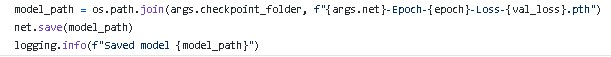# How to test a pytorch model to processing images and videos

hello, i already have a retrained model in pytorch, i used mobilenet-v1-ssd-mp-0_675.pth to retrain with my own image dataset.
After doing this I converted the model to the onnx format to be able to work on the jetson nano, and I managed to process images and videos giving the respective results very well.
My question is how can I process images and videos using the pytorch model (“mb1-ssd-Epoch-20-Loss-1.2040329179303213.pth”), in order to compare the performance between the model on my computer and in my jetson.
I used the jetson-inference repo to do that.
Can someone explain then how do I use the .pth file to get results from images and videos?

Hi,

You have saved your model as a .pth file. The next step is to load the trained model. For this, you do something like:

``````model=Net() ##Your model here
pre_trained_path="path.pth"   ##Your saved model path here
``````

Now, pass image as tensor to the model and get results:

``````with torch.no_grad():
model.eval()
result=model(input_image)
``````

Hope that solves itHi, First thanks for responding.
What am I supposed to put on the model=Net() line? is the path to the model folder, I put the path and got this error message.`raise RuntimeError("{} is a zip archive (did you mean to use torch.load()?)".format(f.name)) RuntimeError: /home/sergio/Documents/jetson-inference/python/training/detection/ssd/models/cars/mb1-ssd-Epoch-49-Loss-0.7622953280806541.pth is a zip archive (did you mean to use torch.load()?)`
And sorry for not realizing much of this, I’m still entering the pytorch now.

Hi,

I think I was not clear. No you dont need to use the model path in `model`.
You must have defined model somewhere. Use that. For perspective, it should look something like this:

``````model=nn.Sequential(
nn.Conv2d(...),
nn.BatchNorm(..),
nn.ReLU()
...
)
``````

or something like this

``````class Net(nn.Module):

def __init__(self):
super(Net, self).__init__()

self.layer1=nn.Linear(...)
self.activate=nn.ReLU()

def forward(self,x):
return self.activate(self.layer1(x))

if __name__=='__main__':
model=Net()
``````

Or maybe if you are using models saved in torchvision, something like:

``````import torchvision.models as M
model=M.mobilenet_v2()
``````

It is not the saved model, but a version of the model which has just been initialized. This is needed as only the weights of the model are saved and in order to restore the model, the actual model skeleton is needed.

On second introspection of the error you are getting, it seems that your .pth file is a zip file and not a weight file. It is highly probable that you didn’t save the model weights in the correct format.

Hi, first thanks for explaining the other problem. Regarding this one I am recording in .pth as you can see here. What will be the problem then? Why does it come in a zip file?I think you do something like this:

``````torch.save(net.state_dict(),model_path)
``````

in place of

``````net.save(model_path)
``````

Thank you very much, I changed the line and it worked very well